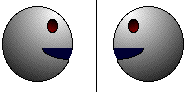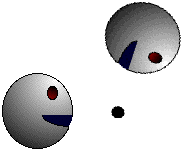Math Central - mathcentral.uregina.caResource Room— glossaries Resource RoomMathematics Glossary - Middle Years Maxine Stinka, Saskatchewan Education, Curriculum and Instruction Note: The definitions included here are those that are used in the Saskatchewan Education document "Mathematics 6-9: A Curriculum Guide for the Middle Level". Various mathematics dictionaries may have different definitions. These definitions are designed to be meaningful to middle level mathematics teachers. Select the first letter of the term that you wish to look for. A | B | C | D | E | F | H | I | J | K | L | M | N | O | P | Q | R | S | T | U | V | W | X | Y | Z R radius (plural: radii) the distance from the centre of a circle to any point on the circle. range in statistics, the difference between the least and the greatest values in a set of data. In more advanced statistics, it is considered the number of values between the greatest and the least, inclusive, and is calculated by the formula range = greatest - least + 1 rate a quotient used to compare two measures in different units e.g. kilometres per hour.   ratio a quotient used to compare two or more quantities of the same units of measure.   rational number see fraction any number that can be written in the form a/b where a and b are integers and b cannot equal 0. It is a negative rational number if it is less than zero i.e. either a or b, but not both, is negative.   ray half a line. i.e.: the portion of a line on one side of a particular point (the endpoint). reciprocals (multiplicative inverses) two numbers whose product is one.   reflection (flip) a transformation of a geometric figure that results in a mirror image of it. The object and the image are equal distance from the line of reflection.reflex angle an angle whose measure is between 1800 and 3600.   regular polygon a polygon with all sides and all angles congruent. An equilateral or equiangular triangle is a regular polygon as is a square. repeating decimal a decimal number that contains a digit or digits that repeat. eg. 1/3 = 0.333... rhombus a quadrilateral with all sides equal in length.   rotation (turn) a transformation of a figure in which the image is formed by turning the figure about a fixed point called the center of rotation which may be inside or outside the figure.Questions or comments about the glossary can be directed to TheCentralizer@MathCentral.uregina.ca or see About Us page for more information.
 Math Central is supported by the University of Regina and the Imperial Oil Foundation.about math central :: site map :: links :: notre site français* Registered trade mark of Imperial Oil Limited. Used under license.